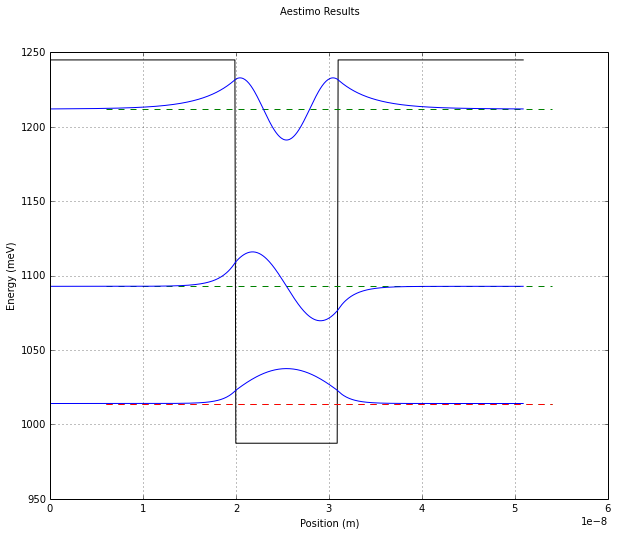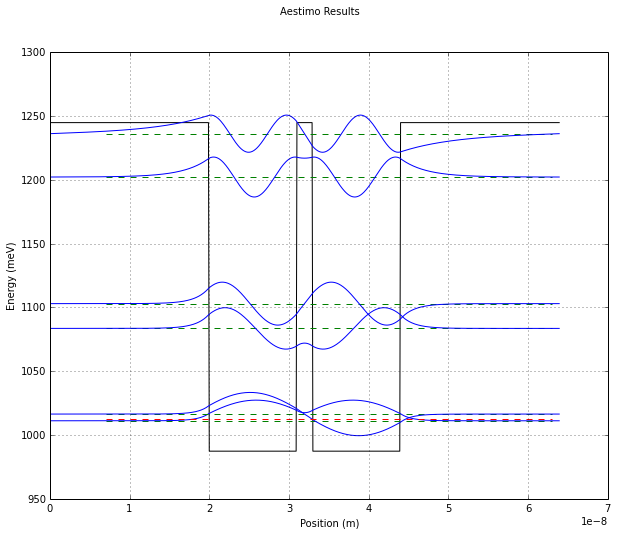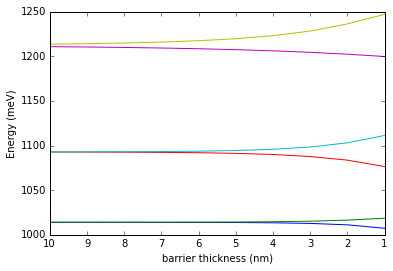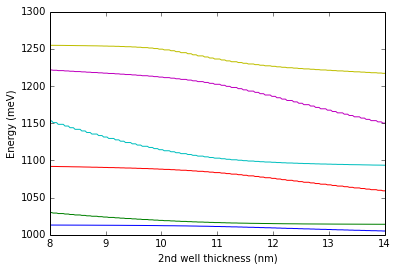# Aestimo Tutorial #2¶

In this tutorial, we are going to consider hybridising of degenerate energy levels. This occurs when two or more systems which share some identical energy levels interact and this interaction causes these energy levels to shift apart from each other. This is called lifting of the degeneracy.

To model this phenomenen, we will model two identical quantum wells as they are brought close together. There is a small problem though, in that the shooting wave method of finding energy levels used by aestimo doesn't work well with degenerate energy levels. So we will have to model the system at the point where the two quantum wells are already starting to interact and their levels hybridise.

First though, we will model a single well on its own.

In :
import aestimo.aestimo as solver
import aestimo.config as ac
ac.messagesoff = True # turn off logging in order to keep notebook from being flooded with messages.
import aestimo.database as adatabase
import numpy as np
import matplotlib.pyplot as plt
import copy
from pprint import pprint

In :
class Structure(object): pass
s0 = Structure() # this will be our datastructure

# TEMPERATURE
s0.T = 15.0 #Kelvin

# COMPUTATIONAL SCHEME
# 0: Schrodinger
# 1: Schrodinger + nonparabolicity
# 2: Schrodinger-Poisson
# 3: Schrodinger-Poisson with nonparabolicity
# 4: Schrodinger-Exchange interaction
# 5: Schrodinger-Poisson + Exchange interaction
# 6: Schrodinger-Poisson + Exchange interaction with nonparabolicity
s0.computation_scheme = 0

# Non-parabolic effective mass function
# 0: no energy dependence
# 1: Nelson's effective 2-band model
# 2: k.p model from Vurgaftman's 2001 paper
s0.meff_method = 0

# Non-parabolic Dispersion Calculations for Fermi-Dirac
s0.fermi_np_scheme = True #needed only for aestimo_numpy2.py

# QUANTUM
# Total subband number to be calculated for electrons
s0.subnumber_e = 3

# APPLIED ELECTRIC FIELD
s0.Fapplied = 0.00 # (V/m)

# GRID
# For 1D, z-axis is choosen
s0.gridfactor = 0.1 #nm
s0.maxgridpoints = 200000 #for controlling the size

# REGIONS
# Region input is a two-dimensional list input.
#         | Thickness (nm) | Material | Alloy fraction | Doping(cm^-3) | n or p type |
s0.material =[
[ 20.0, 'AlGaAs', 0.3, 0.0, 'n'],
[ 11.0, 'GaAs', 0, 2e16, 'n'],
[ 20.0, 'AlGaAs', 0.3, 0.0, 'n'],
]

structure0 = s0

In :
# Initialise structure class
model = solver.StructureFrom(structure0,adatabase) # structure could also be a dictionary.

#calculate QW states
result = solver.Poisson_Schrodinger(model)

%matplotlib inline
#solver.save_and_plot(result,model) # Write the simulation results in files
solver.QWplot(result,figno=None) # Plot QW diagram
solver.logger.info("Simulation is finished.")

Total layer number: 3
INFO:aestimo:Total layer number: 3
Total number of materials in database: 20
INFO:aestimo:Total number of materials in database: 20
calculation time  0.020046 s
INFO:aestimo:calculation time  0.020046 sSimulation is finished.
INFO:aestimo:Simulation is finished.


Now we will model the double QW structure.

In :
s1 = copy.copy(s0) #simpler than redefining everything and changes to s0 should propagate to s1
s1.material = [
[ 20.0, 'AlGaAs', 0.3, 0.0, 'n'],
[ 11.0, 'GaAs', 0, 2e16, 'n'],
[ 2.0, 'AlGaAs', 0.3, 0.0, 'n'], #barrier layer
[ 11.0, 'GaAs', 0, 2e16, 'n'],
[ 20.0, 'AlGaAs', 0.3, 0.0, 'n'],
]
barrier_layer = 2 # defines which layer will be adjusted later
s1.subnumber_e = 6 # There will be double the number of energy states now.

# Initialise structure class
model1 = solver.StructureFrom(s1,adatabase) # structure could also be a dictionary.

#calculate QW states
result1 = solver.Poisson_Schrodinger(model1)

#solver.save_and_plot(result,model)
solver.QWplot(result1,figno=None)
solver.logger.info("Simulation is finished.")

print 'state, Energy'
print '     ,meV'
for num,E in zip(range(result1.subnumber_e),result1.E_state):
print '%5d %7g' %(num,E)

Total layer number: 5
INFO:aestimo:Total layer number: 5
Total number of materials in database: 20
INFO:aestimo:Total number of materials in database: 20
calculation time  0.0383871 s
INFO:aestimo:calculation time  0.0383871 sSimulation is finished.
INFO:aestimo:Simulation is finished.

state, Energy
,meV
0 1011.17
1 1016.44
2  1083.6
3 1103.02
4 1202.29
5 1236.28


We see that the degenerate levels have split in energy. This same thing occurs in atoms and chemical bonds. In solids where there are huge numbers of atoms interacting in this way, there are so many split levels that we talk of energy bands instead of counting the levels directly.

We will now simulate the system for various thicknesses of barriers between the two QWs. The shooting wave algorithm is weak at finding energy levels that are closely spaced and if they are too close together then it will miss both levels. However, the search algorithm can be made to look using any arbitrarily fine grid of energy values if we know that there are energy levels to be found. This will come at the cost of slowing the simulation. In this case, since we are not modelling self-consistant Poisson-Schrodinger effects (which are computationally more expensive due to their iterative nature) then this will not be much of a problem.

In :
q = 1.602176e-19 #C
meV2J=1e-3*q #meV to Joules
# Shooting method parameters for Schrödinger Equation solution
ac.delta_E = 0.005*meV2J #Energy step (Joules) for initial search. Initial delta_E is 1 meV.
#ac.d_E = 1e-5*meV2J #Energy step (Joules) within Newton-Raphson method when improving the precision of the energy of a found level.
#ac.E_start = 0.0    #Energy to start shooting method from (if E_start = 0.0 uses minimum of energy of bandstructure)
#ac.Estate_convergence_test = 1e-9*meV2J

In :
def set_barrier(d):
"""Sets barriers between the two QWs to d (nm)."""
model1.material[barrier_layer] = d
model1.create_structure_arrays() # update the instance's internals

results = []
barriers = np.arange(1,11)
for barrier in barriers:
set_barrier(barrier)
resulti = solver.Poisson_Schrodinger(model1)
results.append(resulti.E_state)

results = np.array(results)

calculation time  1.73137 s
INFO:aestimo:calculation time  1.73137 s
calculation time  1.66888 s
INFO:aestimo:calculation time  1.66888 s
calculation time  1.64143 s
INFO:aestimo:calculation time  1.64143 s
calculation time  1.61989 s
INFO:aestimo:calculation time  1.61989 s
calculation time  1.62761 s
INFO:aestimo:calculation time  1.62761 s
calculation time  1.62602 s
INFO:aestimo:calculation time  1.62602 s
calculation time  1.63055 s
INFO:aestimo:calculation time  1.63055 s
calculation time  1.64965 s
INFO:aestimo:calculation time  1.64965 s
calculation time  1.66582 s
INFO:aestimo:calculation time  1.66582 s
calculation time  1.68194 s
INFO:aestimo:calculation time  1.68194 s


The value of ac.delta_E and the maximum value of the barrier have been chosen so that the all the levels are correctly found but increasing either will show that one or more of the lowest energy levels can easily be lost from the output of the simulation. A reminder that results should always be checked for sanity!

In :
ax1 = plt.subplot(111)
for level in results.transpose(): ax1.plot(barriers,level)
ax1.invert_xaxis()
ax1.set_xlabel("barrier thickness (nm)")
ax1.set_ylabel("Energy (meV)")
plt.show()Here we can how the energy levels split apart as the two quantum wells are brought closer together. This phenomena is often called level repulsion or anti-crossing (or non-crossing or avoided crossing) for quantum mechanical systems. However, it equally occurs with classical oscillators when we study the noraml modes of weakly coupled oscillators.

## Anti-crossing Experiment¶

In many anti-crossing experiments, the frequency of one oscillator is varied so that it crosses the frequency of the other oscillator. We can easily model such an experiment in our case in order to show the type of curves that are often found in the results of such experiments.

In :
#reset delta_E
ac.delta_E = 0.2*meV2J #Energy step (Joules) for initial search. Initial delta_E is 1 meV.

s2 = copy.copy(s0) #simpler than redefining everything and changes to s0 should propagate to s1
s2.material = [
[ 20.0, 'AlGaAs', 0.3, 0.0, 'n'],
[ 11.0, 'GaAs', 0, 2e16, 'n'],
[ 2.0, 'AlGaAs', 0.3, 0.0, 'n'], #barrier layer
[ 9.0, 'GaAs', 0, 2e16, 'n'],
[ 20.0, 'AlGaAs', 0.3, 0.0, 'n'],
]
well2_layer = 3 # defines which layer will be adjusted later
s2.subnumber_e = 6 # There will be double the number of energy states now.

# Initialise structure class
model2 = solver.StructureFrom(s2,adatabase) # structure could also be a dictionary.

def set_well(d):
"""Sets barriers between the two QWs to d (nm)."""
model2.material[well2_layer] = d
model2.create_structure_arrays() # update the instance's internals

# turn off logging
solver.logger.setLevel("WARNING")

#calculate QW states
results2 = []
well_thicknesses = np.linspace(8.0,14.0,200)
for barrier in well_thicknesses:
set_well(barrier)
resulti = solver.Poisson_Schrodinger(model2)
results2.append(resulti.E_state)

results2 = np.array(results2)

Total layer number: 5
INFO:aestimo:Total layer number: 5
Total number of materials in database: 20
INFO:aestimo:Total number of materials in database: 20

In :
ax2 = plt.subplot(111)
for level in results2.transpose(): ax2.plot(well_thicknesses,level)
ax2.set_xlabel("2nd well thickness (nm)")
ax2.set_ylabel("Energy (meV)")
plt.show()We can see how none of the levels cross but instead they seem to exchange characteristics as they pass by each other. If you cover the central part of the graph then this becomes even more obvious. This phenomena occurs in many branches of physics, when coupled oscillators are present. I call this anti-crossing, although apparently it is also called avoided crossing, intended crossing or non-crossing! (see [http://en.wikipedia.org/wiki/Avoided_crossing])